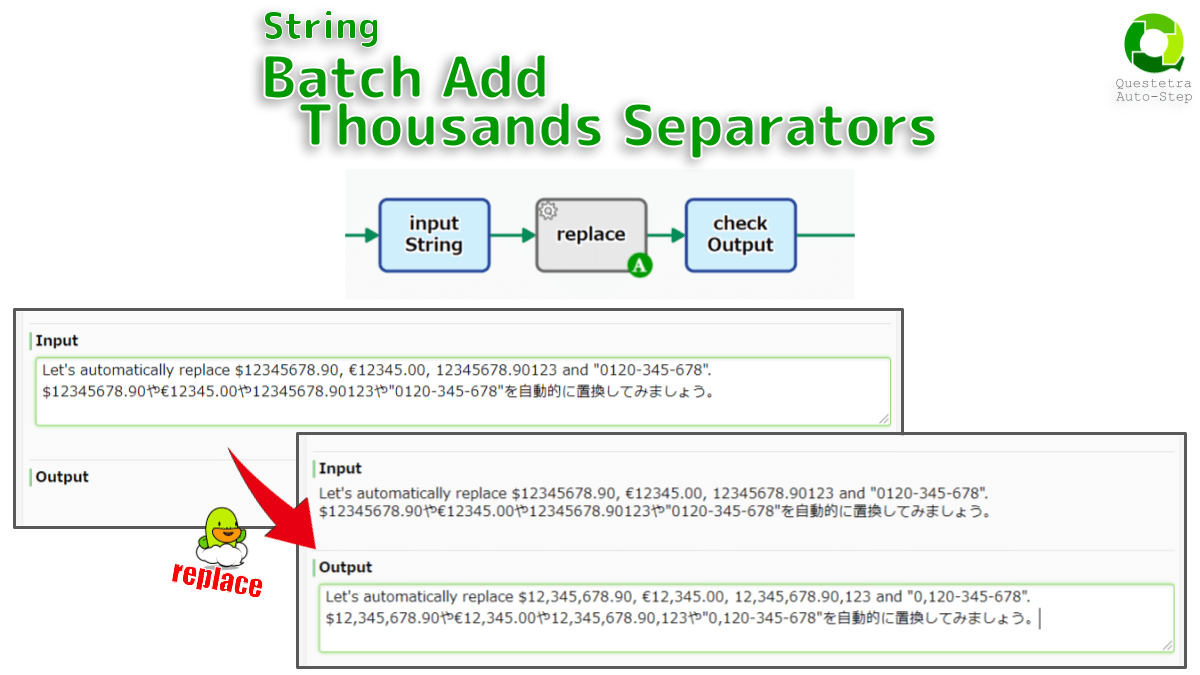Detects numbers with more than three digits in the text and adds thousand separators to each number. A comma, dot, or space can be specified as the digit separator. Note that this replacement will also be applied to decimals, postal codes and years.
Configs
• A: Set String *#{EL}
• B: Select Thousands Separator *
• C: Select STRING DATA that stores Replaced String (update) *
Script (click to open)
``````// GraalJS Script (engine type: 2)

//////// START "main()" /////////////////////////////////////////////////////////////////
main();
function main(){

//// == Config Retrieving / 工程コンフィグの参照 ==
const strInput        = configs.get( "StrConfA" );          /// REQUIRED ///////////
if( strInput      === "" ){
throw new Error( "\n AutomatedTask ConfigError:" +
" Config {A: String} is empty \n" );
}
const strSeparator    = configs.get( "SelectConfB" );       /// REQUIRED ///////////
engine.log( " AutomatedTask Config: Separator: " + strSeparator );
const strPocketOutput = configs.getObject( "SelectConfC" ); /// REQUIRED ///////////

//// == Data Retrieving / ワークフローデータの参照 ==
// (Nothing. Retrieved via Expression Language in Config Retrieving)

//// == Calculating / 演算 ==
let strOutput;
if( strSeparator === "comma" ){
strOutput = strInput.replace(/(\d)(?=(\d{3})+(?!\d))/g, '\$1,'); // supports zh ja
// scripts consistently written without punctuation to separate words
// strOutput = strInput.replace(/\B(?=(\d{3})+(?!\d))/g, ','); // non-word boundary
}else if( strSeparator === "dot" ){
strOutput = strInput // 'dot' sign is used to separate decimal places in JavaScript
.replace(/(\d)\.(?=\d)/g, '\$1,')
.replace(/(\d)(?=(\d{3})+(?!\d))/g, '\$1.');
}else if( strSeparator === "space" ){
strOutput = strInput
.replace(/(\d)\.(?=\d)/g, '\$1,')
.replace(/(\d)(?=(\d{3})+(?!\d))/g, '\$1 ');
}

//// == Data Updating / ワークフローデータへの代入 ==
engine.setData( strPocketOutput, strOutput );

} //////// END "main()" /////////////////////////////////////////////////////////////////

/*
Notes:
- When the process reaches this automated task, the string data will be converted.
- Numeric characters with more than 4 digits (4 characters) will be replaced.
- This would also apply to postal codes, phone numbers, and the year (2022).
- For example, strings such as "Total Report" or "Summary TSV" will be easier to read.

APPENDIX:
JavaScript Numeric literals A decimal point (".")
- Input Sample: `\$12345678.90`
- Output: `\$12,345,678.90`
- Input Sample: "€12345.00`
- Output: `€12,345.00`
- Input Sample: `12345678.90123`
- Output: `12,345,678.90,123` (not good)
- Input Sample: `0120-345-678`
- Output: `0,120-345-678` (not good)
- Implementation of regular expression replacement (comma added)
- `.replace(/(\d)(?=(\d{3})+(?!\d))/g, '\$1,')`
- Replace all "{number}" with "{number},".
- But only if immediately followed by the pattern `(\d{3})+(?!\d)`
- `(\d{3})`: three numeric characters
- `(?!\d)`: non-numeric character
- Implementation of regular expression replacement (period => comma)
- Replace the decimal period (JavaScript literal) with a comma
- `.replace(/(\d)\.(?=\d)/g, '\$1,')`
- Replace all "{number}." will be replaced with "{number},".
- But only if immediately followed by `\d` (Lookahead assertion)
- https://developer.mozilla.org/en-US/docs/Web/JavaScript/Guide/Regular_Expressions/Assertions

Notes-ja:
- 案件がこの自動処理工程に到達した際、文字列（複数行/単一行）を自動的に変換します。
- 4桁以上（4文字以上）の数字文字列が置換されます。
- 郵便番号、電話番号、西暦（2022年）などにも適用されてしまいます。
- たとえば、自動生成された「合計値レポート」や「売上集計TSV」を読みやすくします。

APPENDIX-ja:
- サンプル
- Input: `\$12345678.90`
- Output: `\$12,345,678.90`
- Input: "€12345.00`
- Output: `€12,345.00`
- Input: `12345678.90123`
- Output: `12,345,678.90,123` (not good)
- Input: `0120-345-678`
- Output: `0,120-345-678` (not good)
- 正規表現による置換の実装（カンマ追加）
- 桁区切り記号〔,〕を追加
- `.replace(/(\d)(?=(\d{3})+(?!\d))/g, '\$1,')`
- 全ての『{数字}』を『{数字},』に置換します。
- ただし、その直後に `(\d{3})+(?!\d)` のパターンが続く場合のみ（先読み言明）
- `(\d{3})`: 数字3文字
- `(?!\d)`: 数字以外の文字
- 正規表現による置換の実装（ピリオド⇒カンマ）
- 小数点ピリオド〔JavaScript リテラル〕をカンマに置換
- `.replace(/(\d)\.(?=\d)/g, '\$1,')`
- 全ての『{数字}.』を『{数字},』に置換します。
- https://developer.mozilla.org/ja/docs/Web/JavaScript/Guide/Regular_Expressions/Assertions
*/

``````

2022-01-16 (C) Questetra, Inc. (MIT License)
The Add-on import feature is available with Professional edition.
Freely modifiable JavaScript (ECMAScript) code. No warranty of any kind.

• When the process reaches this automated task, the string data will be automatically converted.
• Numeric characters with more than 4 digits will be replaced.
• This will also apply to postal codes, phone numbers, and the year (2022).
• For example, it makes automatically generated total value reports and sales summary TSVs easier to read.

### Appendix

• Input Sample: `\$12345678.90`
• Output: `\$12,345,678.90`
• Input Sample: “€12345.00`
• Output: `€12,345.00`
• Input Sample: `12345678.90123`
• Output: `12,345,678.90,123` (not good)
• Input Sample: `0120-345-678`
• Output: `0,120-345-678` (not good)
• Implementation of regular expression replacement (comma added)
• Add digit separator `","`.
• `.replace(/(\d)(?=(\d{3})+(?!\d))/g, '\$1,')`
• Replace all “{number}” with “{number},”.
• But only if immediately followed by the pattern `(\d{3})+(?!\d)`
• `(\d{3})`: three numeric characters
• `(?!\d)`: non-numeric character
• Implementation of regular expression replacement (period => comma)